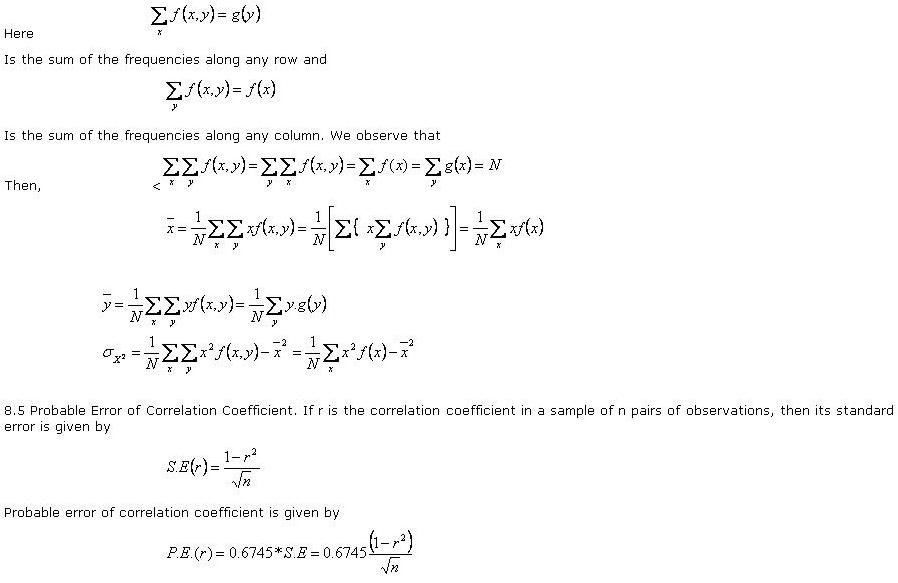# Statistics Assignment Help With Karl Person Coefficient Of Correlation

## 8.3 Karl Person Coefficient Of Correlation:

As a measure of intensity or degree of linear relationship between two variables, karl pearson a British Biometrician, developed a formula called correlation coefficient.

Correlation coefficient between two random variable x and Y, usually denoted by r(X,Y) or simply rXY is a numerical measure of linear relationship between them and is defined as###Probable error is an old measure for testing the reliability of an observed correlation coefficient. The reason for taking the factor 0.6745 is that in a normal distribution, the range µ±0.6745 σ covers 50% of the total area. According to secrist, “the probable error of the correlation co-efficientis an amount which if added to and subtracted from the mean correlation coefficient of correlation from a series selected at random will fall.”

If r< P.E>(r). correlation is not at all significant. If r> 6P.E.(r), it is definitely significant. A rigorous method of testing the significance of an observed correlation coefficient will be discussed later in”test of significance” in sampling.

Probable error also enables us to find the limits within which the population correlation can be expected to vary. The limits are r±P.E.(r).